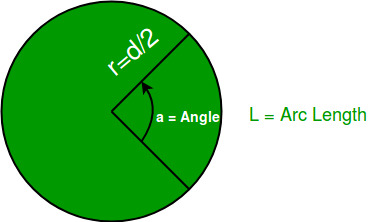# Arc length from given Angle

• Last Updated : 20 Jun, 2022

An angle is a geometrical figure when two rays meet at a common point on a plane. These rays form the sides of the angle and the meeting point is referred as the vertex of the angle. There is something that we need to keep in mind that the plane that forms an angle doesn’t need to be a Euclidean plane. Now, in a circle, the length of an arc is a portion of the circumference. The figure explains the various parts we have discussed:Given an angle and the diameter of a circle, we can calculate the length of the arc using the formula:

```ArcLength = ( 2 * pi * radius ) * ( angle / 360 )
Where pi = 22/7,
diameter = 2 * radius,
angle is in degree.```

Examples :

```Input :
Diameter = 25
Angle = 45
Explanation : ((22/7) * 25) * (45/360)
Output : 9.821 (rounded)

Input :
Diameter = 80
Angle = 60
Explanation : ((22/7) * 80) * (60/360)
Output : 41.905 (rounded)```

Note: If angle is greater than or equal to 360 degree, then the arc length cannot be calculated, since no angle is possible.

## C++

 `// C++ program to calculate``// length of an arc``#include ``using` `namespace` `std;` `// function to calculate``// arc length``double` `arcLength(``double` `diameter,``                 ``double` `angle)``{``    ``double` `pi = 22.0 / 7.0;``    ``double` `arc;` `    ``if` `(angle >= 360)``    ``{``        ``cout<< ``"Angle cannot"``,``               ``" be formed"``;``        ``return` `0;``    ``}``    ``else``    ``{``        ``arc = (pi * diameter) *``              ``(angle / 360.0);``        ``return` `arc;``    ``}``}` `// Driver Code``int` `main()``{``    ``double` `diameter = 25.0;``    ``double` `angle = 45.0;``    ` `    ``double` `arc_len = arcLength(diameter,``                                 ``angle);``    ``cout << (arc_len);` `    ``return` `0;``}`

## Java

 `// Java program to calculate``// length of an arc``public` `class` `Arc {``    ` `    ``// function to calculate arc length``    ``static` `double` `arcLength(``double` `diameter,``                              ``double` `angle)``    ``{``        ``double` `pi = ``22.0` `/ ``7.0``;``        ``double` `arc;` `        ``if` `(angle >= ``360``) {``            ``System.out.println(``"Angle cannot"``                              ``+ ``" be formed"``);``            ``return` `0``;``        ``}``        ``else` `{``            ``arc = (pi * diameter) * (angle / ``360.0``);``            ``return` `arc;``        ``}``    ``}``    ` `    ``// Driver Code``    ``public` `static` `void` `main(String args[])``    ``{``        ``double` `diameter = ``25.0``;``        ``double` `angle = ``45.0``;``        ``double` `arc_len = arcLength(diameter, angle);``        ``System.out.println(arc_len);``    ``}``}`

## Python3

 `# Python3 code to calculate``# length of an arc``import` `math` `# function to calculate arc length``def` `arcLength(diameter, angle ):``    ``if` `angle >``=` `360``:``        ``print``(``"Angle cannot be formed"``)``        ``return` `0``    ``else``:``        ``arc ``=` `(``3.142857142857143` `*` `diameter) ``*` `(angle ``/` `360.0``)``        ``return` `arc``        ` `# Driver Code``diameter ``=` `25.0``angle ``=` `45.0``arc_len ``=` `arcLength(diameter, angle)``print``(arc_len)` `# This code is contributed by "Sharad_Bhardwaj".`

## C#

 `// C# program to calculate length of an arc``using` `System;` `public` `class` `GFG {``    ` `    ``// function to calculate arc length``    ``static` `double` `arcLength(``double` `diameter,``                              ``double` `angle)``    ``{``        ``double` `pi = 22.0 / 7.0;``        ``double` `arc;` `        ``if` `(angle >= 360) {``            ``Console.WriteLine(``"Angle cannot"``                              ``+ ``" be formed"``);``            ``return` `0;``        ``}``        ``else` `{``            ``arc = (pi * diameter) * (angle / 360.0);``            ``return` `arc;``        ``}``    ``}``    ` `    ``// Driver Code``    ``public` `static` `void` `Main()``    ``{``        ` `        ``double` `diameter = 25.0;``        ``double` `angle = 45.0;``        ` `        ``double` `arc_len = arcLength(diameter, angle);``        ` `        ``Console.WriteLine(arc_len);``    ``}``}` `// This code is contributed by Anant Agarwal.`

## PHP

 `= 360)``        ``{``            ``echo` `"Angle cannot"``,``                   ``" be formed"``;``            ``return` `0;``        ``}``        ``else``        ``{``            ``\$arc` `= (``\$pi` `* ``\$diameter``) *``                   ``(``\$angle` `/ 360.0);``            ``return` `\$arc``;``        ``}``    ``}``    ` `// Driver Code``\$diameter` `= 25.0;``\$angle` `= 45.0;``\$arc_len` `= arcLength(``\$diameter``, ``\$angle``);``echo` `(``\$arc_len``);` `// This code is contributed by ajit``?>`

## Javascript

 ``

Output:

`9.821428571428571`

Time Complexity: O(1)
Auxiliary Space: O(1)

This article is contributed by Chinmoy Lenka. If you like GeeksforGeeks and would like to contribute, you can also write an article using write.geeksforgeeks.org or mail your article to review-team@geeksforgeeks.org. See your article appearing on the GeeksforGeeks main page and help other Geeks.
Please write comments if you find anything incorrect, or you want to share more information about the topic discussed above.

My Personal Notes arrow_drop_up